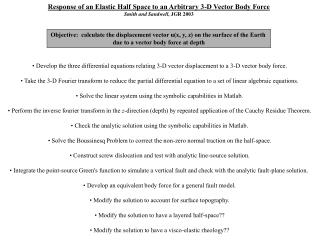# Response of an Elastic Half Space to an Arbitrary 3-D Vector Body Force - PowerPoint PPT PresentationDownload PresentationResponse of an Elastic Half Space to an Arbitrary 3-D Vector Body Force

Response of an Elastic Half Space to an Arbitrary 3-D Vector Body ForceDownload Presentation## Response of an Elastic Half Space to an Arbitrary 3-D Vector Body Force

- - - - - - - - - - - - - - - - - - - - - - - - - - - E N D - - - - - - - - - - - - - - - - - - - - - - - - - - -
##### Presentation Transcript

1. Response of an Elastic Half Space to an Arbitrary 3-D Vector Body Force Smith and Sandwell, JGR 2003 • Develop the three differential equations relating 3-D vector displacement to a 3-D vector body force. • Take the 3-D Fourier transform to reduce the partial differential equation to a set of linear algebraic equations. • Solve the linear system using the symbolic capabilities in Matlab. • Perform the inverse fourier transform in the z-direction (depth) by repeated application of the Cauchy Residue Theorem. • Check the analytic solution using the symbolic capabilities in Matlab. • Solve the Boussinesq Problem to correct the non-zero normal traction on the half-space. • Construct screw dislocation and test with analytic line-source solution. • Integrate the point-source Green's function to simulate a vertical fault and check with the analytic fault-plane solution. • Develop an equivalent body force for a general fault model. • Modify the solution to account for surface topography. • Modify the solution to have a layered half-space?? • Modify the solution to have a visco-elastic rheology?? Objective: calculate the displacement vector u(x, y, z) on the surface of the Earth due to a vector body force at depth

2. Full Displacement Solution: (Source) (Image) (Boussinesq) Components:

3. Sketch of 3-D fault in an elastic half-space Force couple • Magnitude ~ slip rate • Direction || to plate motion User defines: d1, d2, zobs, x1, x2, y1, y2, and F • Analytic form of the force couple is the derivative of a Gaussian function with half-width equal to cell spacing • Cosine transform in x-direction is used for constant velocity difference across the plate boundary • Uniform far-field velocity is simulated by arranging the fault trace to be cyclic in the y-direction

4. San Andreas Fault Segments Assign slip rates from literature Paralleling segments: sum to 40 mm/yr Locking depths? Use 1099 horizontal GPS velocity measurements to solve for locking depth

5. Locking Depth Results rms model misfit: 2.43 mm/yr

6. Predicted Vertical Uplift Geodetic estimates Geologic estimates San Gabriel Mts 3-10mm/yr [Brown, 1991] San Bernadino Mts 2 mm/yr [Yule and Seih, 1997]

7. Coulomb Stress